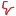# The Implications of Arithmetic

This is just a random thought, but interesting to ponder. My recollection of learning arithmetic is as follows…

1. First I learned to count.
2. Then I learned addition because it’s basically like counting only slightly more complicated.
3. Then I learned subtraction because it was like addition only backwards (or counting backwards). Note the I didn’t learn about negative numbers until later.
4. Then I learned about multiplication because it was basically addition over and over again. Exponentiation or multiplication over and over again, of course, came much later.
5. Finally, for basic arithmetic, I learned about division because it was clearly more complicated and mysterious.

There’s a danger of getting causality reversed here, but I’m going to take a chance. I wonder if there are implications of learning addition first, subtraction second and multiplication and division being that advanced stuff. Addition, in particular, creates a bias for linear, open-ended systems. These, of course, don’t exist in reality but they can be good approximations for narrow views of larger systems. In other words, they work for awhile but eventually cease to be an accurate reflection of our observations as subtler forces begin to assert themselves on the system of interest.

This way of thinking may be easiest for us partly because we’ve been taught that it’s easiest for us. What if we inverted how we learned arithmetic. For example …

1. First learn to partition. Put all the toys on the floor and divide them up. Notice that each of the new piles is smaller than the original pile.
2. Teach division first. This may require coming up with a new notation, but that would require more thought. It’s interesting how linear, open ended assumptions are built into at least simple arithmetical notation.
3. Teach multiplication next, perhaps rapidly including exponentiation.
4. Finally teach subtraction and addition as exceptional use cases on top of division and multiplication.

Since we weren’t raised this way it’s a little hard to wrap our heads around, but what impact might this have on our world views? We would certainly have a greater appreciation for bounded systems. Depending on how we moved from division to multiplication, we might have a better appreciation for non-linear systems. Our accounting systems might be dramatically different and do a better job of reflecting the facts that we experience over larger spaces and time frames.

It would be interesting to know if humans have experimented with this in various isolated and possibly extinct cultures. I don’t know of any at the moment, but if you do please let me know.

Likes(0)Dislikes(0)